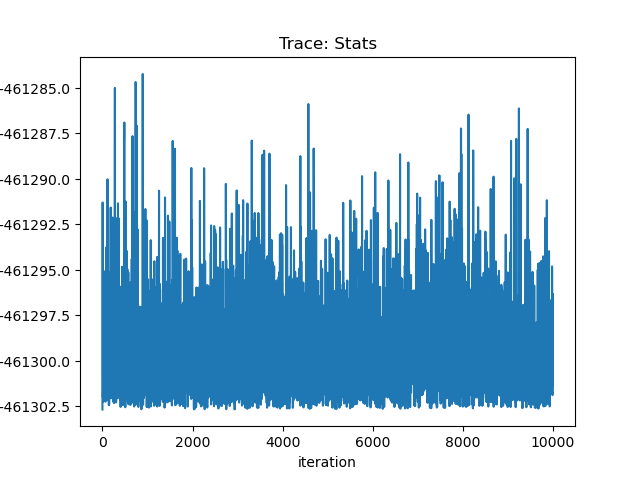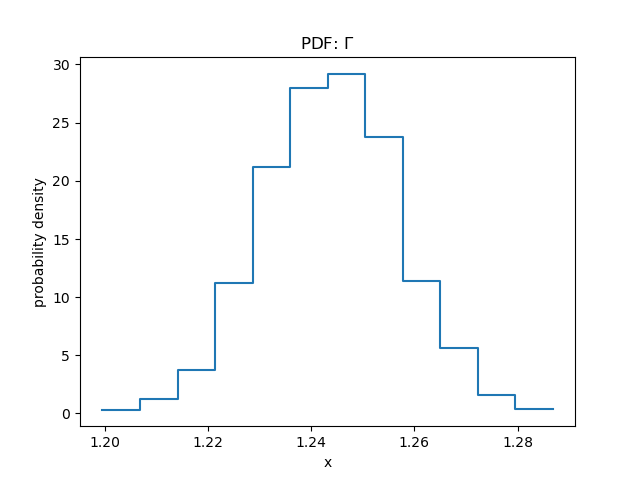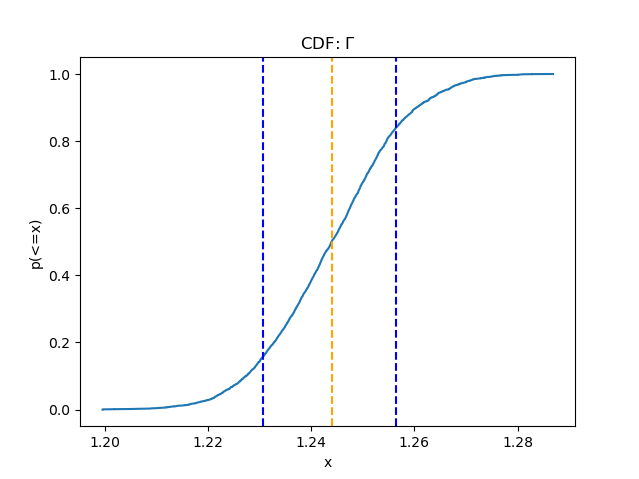# Bayesian Analysis in SherpaSherpa Threads (CIAO 4.11 Sherpa v1)

## Overview

#### Synopsis:

pyBLoCXS is a sophisticated Markov chain Monte Carlo (MCMC) based algorithm designed to carry out Bayesian Low-Count X-ray Spectral (BLoCXS) analysis in the Sherpa environment. The algorithm may be used to explore parameter space at a suspected minimum using a pre-defined Sherpa model to high-energy X-ray spectral data.

Use this thread if you are interested in conducting Bayesian Low-Counts X-ray Spectral (BLoCXS) analysis in the Sherpa environment.

Last Update: 13 Dec 2018 - reviewed for CIAO 4.11, no content change.

## Introduction to pyBLoCXS

The pyBLoCXS code is a Python extension to Sherpa that explores model parameter space at a suspected minimum using a pre-defined Sherpa model to high-energy X-ray spectral data. pyBLoCXS includes a flexible definition of priors and can efficiently probe the model parameter space to characterize the posterior distributions. It allows for variations in the calibration information, and can be used to compute posterior predictive p-values for the likelihood ratio test (see Protassov, et al. 2002, ApJ 571, 542, Statistics, Handle with Care: Detecting Multiple Model Components with the Likelihood Ratio Test).

The Sherpa get_draws function runs a pyBLoCXS chain using fit information associated with the user-specified data set(s) and the currently set sampler and parameter priors, for a specified number of iterations. It returns an array of simulated fit statistic values, an array of True or False accepted/rejected Booleans, and a 2-D array of associated model parameter values.

A general description of the MCMC techniques employed by the pyBLoCXS algorithm can be found in the following references:

The Sherpa pyBLoCXS functions include:

## Getting Started

Please follow the Obtaining Data Used in Sherpa thread in order to obtain the sample data used in this thread. The spectral data products for the PKS 1127-145 quasar core are located in the pyblocxs sub-directory.

## Fitting a Model to the Spectrum

The pyBLoCXS routine requires information about a fit to a data set in order to run; therefore, we begin by loading a source spectrum into Sherpa, fitting a model to it, and calculating confidence intervals on thawed parameters using the covariance method.

The source spectrum is loaded with load_pha, and the source model expression to be used for fitting is defined with set_model. We decide to restrict the analysis to the 0.5-7.0 keV energy range, using the ignore command.

```sherpa> load_pha(1, "core.pi")
sherpa> set_model(xsphabs.abs0*powlaw1d.p1)
sherpa> ignore(None,0.5)
sherpa> ignore(7.,None)
```

We fit an absorbed power-law model to the data using the robust, Nelder-Mead Simplex fit optimization method and Cash fit statistic in order to select a Poisson likelihood as appropriate for pyBLoCXS simulations. Then, we estimate confidence intervals on all thawed parameters with the Sherpa covariance command.

```sherpa> set_method("neldermead")
sherpa> set_stat("cash")

sherpa> fit()
WARNING: data set 1 has associated backgrounds, but they have not been subtracted, nor have background models been set
Dataset               = 1
Statistic             = cash
Initial fit statistic = 1.0011e+08
Final fit statistic   = -461303 at function evaluation 623
Data points           = 444
Degrees of freedom    = 441
Change in statistic   = 1.00571e+08
abs0.nH        0.0674479
p1.gamma       1.24121
p1.ampl        0.00067359

sherpa> covar()
WARNING: data set 1 has associated backgrounds, but they have not been subtracted, nor have background models been set
Dataset               = 1
Confidence Method     = covariance
Iterative Fit Method  = None
Statistic             = cash
covariance 1-sigma (68.2689%) bounds:
Param            Best-Fit  Lower Bound  Upper Bound
-----            --------  -----------  -----------
abs0.nH         0.0674479  -0.00426058   0.00426058
p1.gamma          1.24121   -0.0124818    0.0124818
p1.ampl        0.00067359 -9.02561e-06  9.02561e-06
```

## Running the pyBLoCXS Routine

pyBLoCXS simulations are executed with the get_draws function, using the fit information associated with our specified data set(s)—and the current sampler and parameter priors—for a specified number of iterations. The simplest case uses the default MetropolisMH sampler, and flat priors for all parameters.

The list of all available samplers is returned by the list_samplers function, and the currently set sampler may be viewed with get_sampler_name, as demonstrated below.

```sherpa> print(get_sampler_name())
MetropolisMH

sherpa> list_samplers()
['fullbayes', 'pragbayes', 'mh', 'metropolismh']
```

The sampler—the type of jumping rule to be used in MCMC—can be either MH, MetropolisMH, pragbayes, or fullbayes (note that fullbayes is untested, new functionality and not recommended at this time). Metropolis and Metropolis-Hastings are the two standard algorithms for sampling the model parameter space (the posterior). Metropolis jumps to the new set of parameters from the current parameter set, while Metropolis-Hastings jumps always from the best fit. The Sherpa sampler MH is a pure Metropolis-Hastings, which always jumps from the best-fit. MetropolisMH is a mixture of Metropolis and Metropolis-Hastings, selected with a probability p_M. PragBayes is used when the effective area calibration uncertainty is to be included in the calculation.Note

At each nominal MCMC iteration, a new calibration product is generated, and a series of N (option in set_sampler_opt) MCMC sub-iteration steps are carried out, choosing between Metropolis and Metropolis-Hastings types of samplers with probability p_M (option in set_sampler_opt). Only the last of these sub-iterations are kept in the chain.

The get_sampler function is available for accessing the default settings of the current sampler, and set_sampler_opt for modifying those settings.

```sherpa> get_sampler()
{'log': False, 'priors': (), 'defaultprior': True, 'scale': 1, 'originalscale': True, 'priorshape': False, 'inv': False, 'p_M': 0.5, 'sigma_m': False}
```

We accept the default settings for the initial, simplest case and run the sampler using the get_draws function, specifying the names of the output arrays and the number of iterations.

```stats, accept, params = get_draws(niter=1e4)
```

Here we use Python syntax to name the data arrays created by the simulations: stats, accept, and params. stats contains a 1-D array of floating-point statistic values; accept contains a 1-D array of True or False accepted/rejected Booleans for each iteration; and params contains a N-D array of parameter values, where N is the number of free parameters in the model. In this example, N is equal to 3.

The get_draws command above produces the following screen output, showing which parameters are being sampled and what prior function is assumed for the simulation:

```Using Priors:
abs0.nH: <function flat at 0x16a43f50>
p1.gamma: <function flat at 0x16a43f50>
p1.ampl: <function flat at 0x16a43f50>
```

## Visualizing the Results of the pyBLoCXS Simulations

There are several Sherpa plotting functions available for visualizing statistics and parameter values for each accepted iteration; these include:

### Fit Statistic Values

The plot_trace function can be used to plot by default the entire array of statistic values for each sample. We can use the associated get_trace_plot command to specify plot preferences, such as the desired value for line thickness, and then enter the name of the fit statistic array returned by get_draws into the plot_trace expression (stats) to create the plot. Finally, we can save the resulting plot to a PNG format image file with print_window.

```sherpa> get_trace_plot().plot_prefs
{'errcolor': None,
'errstyle': None,
'errthickness': None,
'linecolor': 'red',
'linestyle': 1,
'linethickness': 3,
'ratioline': False,
'symbolcolor': None,
'symbolfill': True,
'symbolsize': None,
'symbolstyle': 0,
'xaxis': False,
'xerrorbars': False,
'xlog': False,
'yerrorbars': False,
'ylog': False}

sherpa> get_trace_plot().plot_prefs["linethickness"] = 1
sherpa> plot_trace(stats,name="Stats")

sherpa> print_window("trace_stats.png")
```

### Figure 1: Trace Plot of Fit Statistic Values### Figure 1: Trace Plot of Fit Statistic Values

We can also choose to filter the fit statistic array and plot only a subset of the iterations—for example, elements 8000-9000—as well as plot only positive statistic values (Cash is defined as a negative value of the likelihood).

```sherpa> plot_trace(-stats[8000:9000],name="Stats")
```

### Figure 2: Trace Plot of Filtered Statistic Values### Model Parameter Values

We may also examine the distribution of model parameters resulting from the simulations, stored in the params array returned by get_draws. Like the other data arrays output by get_draws, this array is a standard NumPy ndarray, which means we can obtain information about the array using Python syntax, for example:

```sherpa> params.shape
(3, 10001)

sherpa> params.dtype
dtype('float64')

sherpa> params.size
30003
```

By manipulating the params array in this way, we can access the simulation results for any of the three model parameters sampled. Below, we filter params to return all values for the first parameter, abs0.nH, and then again to return just the values for the tenth through twentieth iterations.

```sherpa> params[0,:]

array([ 0.06744795,  0.06461282,  0.06461282, ..., 0.06796692,  0.06511419,  0.06511419,  0.06898862,  0.06663478, 0.06482555])

sherpa> params[0,10:20]

array([ 0.06441612,  0.0644731 ,  0.06021192,  0.06021192,  0.06788906,
0.06727158,  0.06727158,  0.06727158,  0.06890315,  0.06890315])
```

Likewise, we can list the parameter values for the second parameter, p1.gamma, for iterations 1000-1020:

```sherpa> params[1,1010:1020]

array([ 1.25812098,  1.24119548,  1.24933646,  1.23620416,  1.24500267,
1.22458986,  1.22458986,  1.22458986,  1.24475196,  1.23661198])
```

The probability distribution of parameter values may be plotted with the plot_pdf command, as shown below for the gamma parameter:

```sherpa> plot_pdf(params[1,:],name=r"\Gamma")
```

### Figure 3: Probability Distribution of a Selected Parameter### Figure 3: Probability Distribution of a Selected Parameter

The plot_cdf function displays the cumulative distribution, with the median and 1σ bounds marked on the plot:

```sherpa> plot_cdf(params[1,:],name=r"\Gamma")
```

### Figure 4: Cumulative Distribution of a Selected Parameter### Figure 4: Cumulative Distribution of a Selected Parameter

To access all information about the displayed distribution, the get_pdf_plot and get_cdf_plot functions may be used; for example:

```sherpa> print(get_cdf_plot())
points = [ 1.1969  1.1985  1.1986 ...,  1.2811  1.2834  1.2893]
x      = [  9.9990e-05   1.9998e-04   2.9997e-04 ...,   9.9980e-01   9.9990e-01
1.0000e+00]
y      = [ 1.2412  1.2575  1.2575 ...,  1.2492  1.2447  1.2447]
median = 1.24145700495
lower  = 1.22858082704
upper  = 1.25370753547
xlabel = x
ylabel = p(<=x)
title  = CDF: \Gamma

plot_prefs = {'linethickness': 3, 'symbolcolor': None, 'symbolfill': True, 'xlog': False, 'ylog': False, 'errthickness': None, 'yerrorbars': False, 'linecolor': 'red', 'errstyle': None, 'linestyle': 1, 'symbolstyle': 0, 'errcolor': None, 'xaxis': False, 'ratioline': False, 'xerrorbars': False, 'symbolsize': None}

```

To access the values for the median of the distribution, and the lower and upper bounds, we can use the function directly, as follows:

```sherpa> get_cdf_plot().median
1.2410612917551687

sherpa> get_cdf_plot().lower
1.2283699914156427

sherpa> get_cdf_plot().upper
1.2543167774241046
```

We can also display the values of the parameters in a scatter plot, showing correlations between different parameters. Here we display abs0.nH and p1.gamma, showing parameter correlation typical of an X-ray data set.

```sherpa> plot_scatter(params[0,:], params[1,:], name = r"nH vs. \Gamma", xlabel = "nH", ylabel =r"\Gamma")
```

### Figure 5: Scatter plot of Two Correlated Model Parameters### Figure 5: Scatter plot of Two Correlated Model Parameters

Finally, we can access the data arrays and preferences defining the scatter plot with the get_scatter_plot function:

```sherpa> print(get_scatter_plot())
x      = [ 0.0674  0.0646  0.0646 ...,  0.069   0.0666  0.0648]
y      = [ 1.2412  1.2463  1.2463 ...,  1.2548  1.2422  1.2311]
yerr   = None
xerr   = None
xlabel = NH
ylabel = \Gamma
title  = Scatter: NH vs. \Gamma
plot_prefs = {'linethickness': None, 'symbolcolor': None, 'symbolfill': True, 'xlog': False, 'ylog': False, 'errthickness': None, 'yerrorbars': False, 'linecolor': None, 'errstyle': 'line', 'linestyle': 0, 'symbolstyle': 4, 'errcolor': None, 'xaxis': False, 'ratioline': False, 'xerrorbars': False, 'symbolsize': 1}
```

## Scripting It

The file, fit.py , performs the primary commands used above. It can be executed by typing 'exec(open("fit.py").read())' on the Sherpa command line. The Sherpa script command may be used to save everything typed on the command line in a Sherpa session:

```sherpa> script(filename="sherpa.log", clobber=False)
```

(Note that restoring a Sherpa session from such a file could be problematic since it may include syntax errors, unwanted fitting trials, et cetera.)

The CXC is committed to helping Sherpa users transition to new syntax as smoothly as possible. If you have existing Sherpa scripts or save files, submit them to us via the CXC Helpdesk and we will provide the CIAO/Sherpa 4.11 syntax to you.

## History

 15 Dec 2011 new for CIAO 4.4 11 Dec 2013 reviewed for CIAO 4.6: no changes 19 Mar 2015 updated for CIAO 4.7, no content change. 09 Dec 2015 reviewed for CIAO 4.8, no content change. 01 Dec 2016 reviewed for CIAO 4.9, no content change. 29 May 2018 reviewed for CIAO 4.10, no content change. 13 Dec 2018 reviewed for CIAO 4.11, no content change.